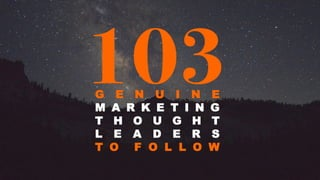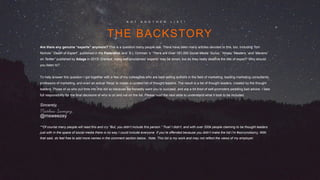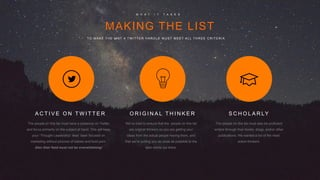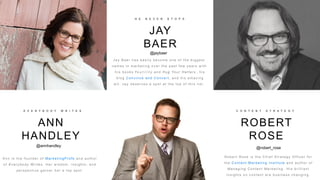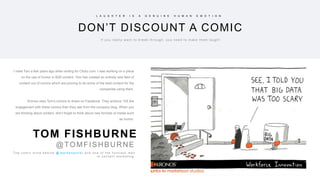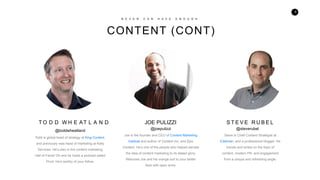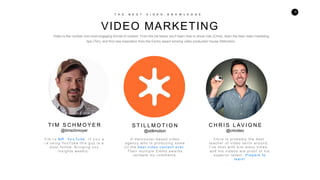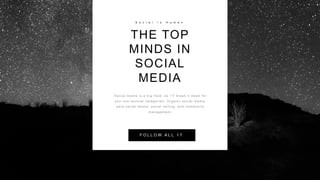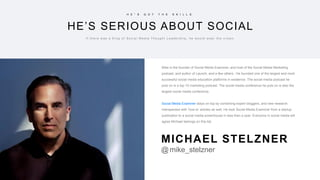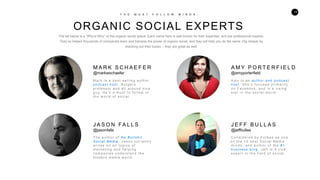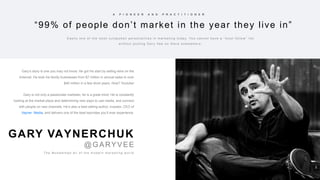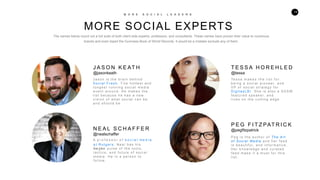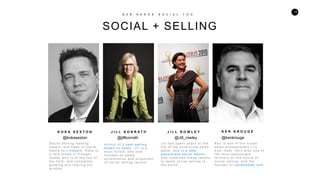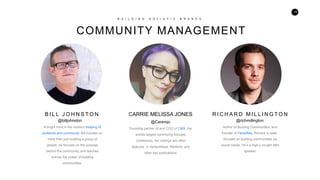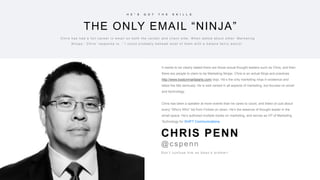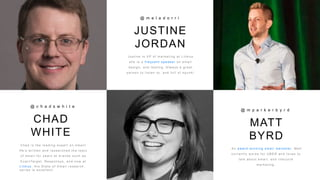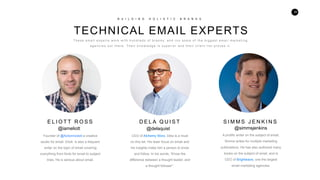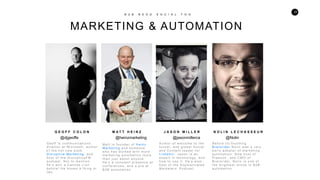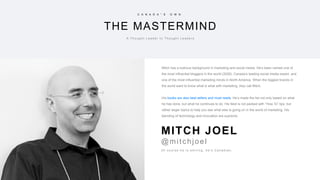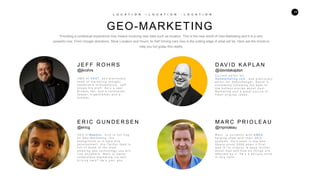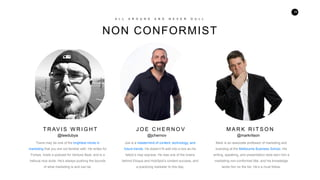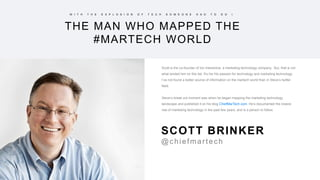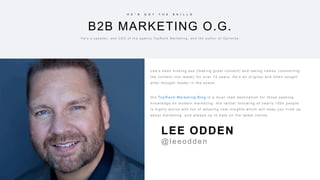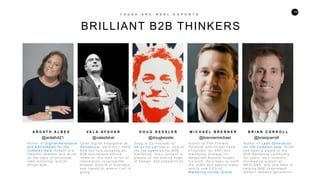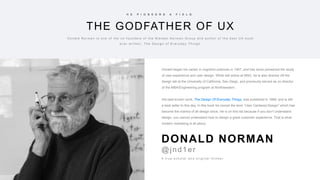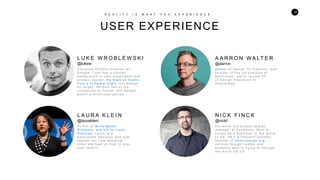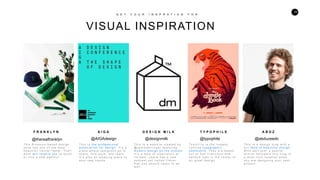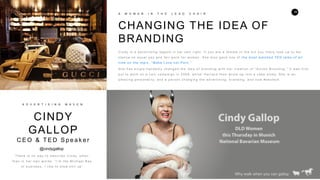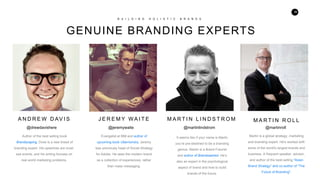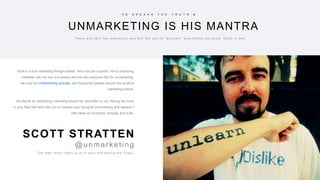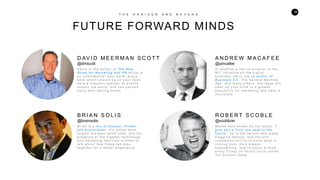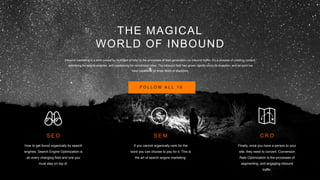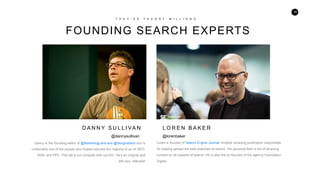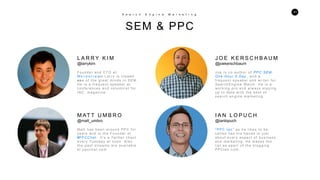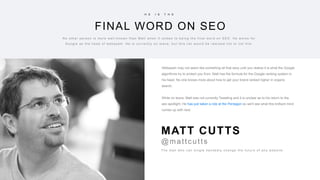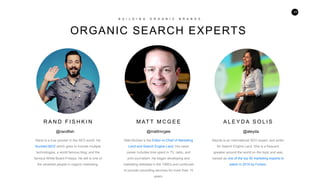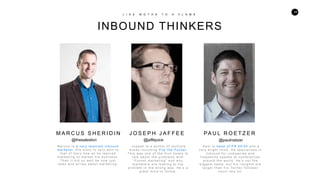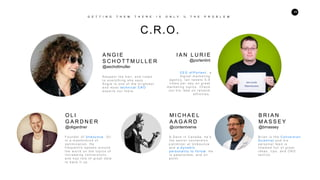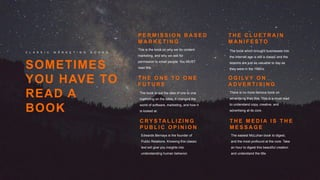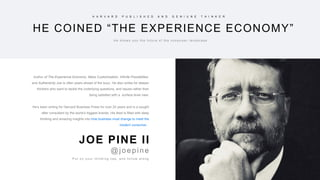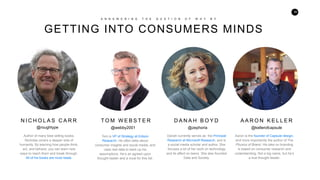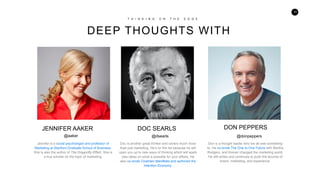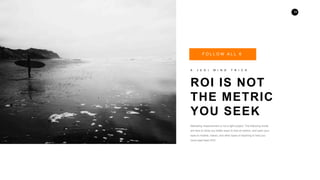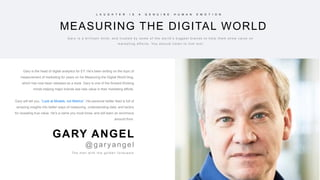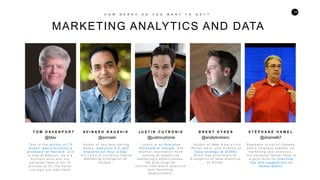1 of 55

### 103 Genuine Marketing Thought Leaders

1. 1 103G E N U I N E M A R K E T I N G T H O U G H T L E A D E R S T O F O L L O W
3. 3 MAKING THE LIST W H A T I T T A K E S TO M A K E T H E L I S T A T W I T T E R H A N D L E M U S T M E E T A L L T H R E E C R I T E R I A The people on this list must have a presence on Twitter and focus primarily on the subject at hand. This will keep your “Thought Leadership” feed laser focused on marketing without pictures of babies and food porn. Also their feed must not be overwhelming! A C T I V E O N T W I T T E R We’ve tried to ensure that the people on this list are original thinkers so you are getting your ideas from the actual people having them, and that we’re putting you as close as possible to the best minds out there. O R I G I N A L T H I N K E R The people on this list must also be proficient writers through their books, blogs, and/or other publications. We wanted a list of the most active thinkers. S C H O L A R LY
4. 4 HOW TO USE THE LIST I n s t r u c t i o n s T h e l i s t i s s e g m e n t e d b y c a t e g o r y. E a c h c a t e g o r y i s a l s o a Tw i t t e r l i s t . Yo u ’ l l f i n d t h e l i n k t o t h e l i s t a t t h e b e g i n n i n g o f e a c h s e c t i o n . F o l l o w t h e m a n d y o u i n s t a n t l y h a v e a c o n t i n u o u s f e e d o f s o m e o f t h e b e s t c o n t e n t . O r c h o o s e t o f o l l o w a l l 1 0 3 i n s t a n t l y b y s i m p l y c l i c k i n g t h e b u t t o n b e l o w. F O L L O W A L L 1 0 3 T h e l i s t i s a l s o h e r e t o h e l p i n t r o d u c e y o u t o n e w d i s c i p l i n e s o f m a r k e t i n g , a n d n e w t h o u g h t l e a d e r s y o u m a y n o t f i n d o n y o u r o w n . C L I C K H E R E T O A D D T H E F U L L L I S T T O Y O U R T W I T T E R A C C O U N T
5. 5 THE PEOPLE CHANGING THE IDEA OF CONTENT C O N T E N T M A R K E T I N G Content marketing is a massive term, so we’ve decided to break this section down in the most progressive minds in content. The 11 people we’ve chosen will give you a wide range of ideas and best practices covering traditional ideas of content, and brand new ones as well. F O L L O W A L L 1 0
6. 6 ANN HANDLEY E V E R Y B O D Y W R I T E S A n n i s t h e f o u n d e r o f M a r k e t i n g P r o f s and author o f E v e r y b o d y W r i t e s . Her wisdom, insights, and p e r s p e c t i v e g a r n e r h e r a t o p s p o t . JAY BAER H E N E V E R S T O P S ROBERT ROSE C O N T E N T S T R A T E G Y @annhandley @jaybaer J a y B a e r h a s e a s i l y b e c o m e o n e o f t h e b i g g e s t n a m e s i n m a r k e t i n g o v e r t h e p a s t f e w y e a r s w i t h h i s b o o k s Yo u t i l i t y a n d Hug Your Hatters , his b l o g C o n v i n c e a n d C o n v e r t , a n d h i s a m a z i n g w i t . J a y d e s e r v e s a s p o t a t t h e t o p o f t h i s l i s t . @robert_rose R o b e r t R o s e i s t h e C h i e f S t r a t e g y O f f i c e r f o r the Content Marketing Institute and author of M a n a g i n g C o n t e n t M a r k e t i n g . His brilliant insights on content are business changing.
7. 7 DON’T DISCOUNT A COMIC L A U G H T E R I S A G E N U I N E H U M A N E M O T I O N I f y o u r e a l l y w a n t t o b r e a k t h r o u g h , y o u n e e d t o m a k e t h e m l a u g h ! I meet Tom a few years ago when writing for Clickz.com. I was working on a piece on the use of humor in B2B content. Tom has created an entirely new field of content out of comics which are proving to be some of the best content for the companies using them. Kronos uses Tom’s comics to share on Facebook. They achieve 10X the engagement with these comics than they see from the company blog. When you are thinking about content, don’t forget to think about new formats of media such as humor. T h e c o m i c m i n d b e h i n d @ m a r k e t o o n i s t a n d o n e o f t h e f u n n i e s t m e n i n c o n t e n t m a r k e t i n g TOM FISHBURNE @TOMFISHBURNE
8. 8 CONTENT (CONT) N E V E R C A N H A V E E N O U G H Steve is Chief Content Strategist at Edelman, and a professional blogger. He travels and writes on the topic of content, modern PR, and engagement from a unique and refreshing angle. S T E V E R U B E LT O D D W H E AT L A N D @toddwheatland Todd is global head of strategy at King Content, and previously was head of marketing at Kelly Services. He’s also in the content marketing Hall of Fame! Oh and he hosts a podcast called Pivot. He’s worthy of your follow. JOE PULIZZI @joepulizzi Joe is the founder and CEO of Content Marketing Institute and author of Content Inc, and Epic Content. He’s one of the people who helped elevate the idea of content marketing to its stated glory. Welcome Joe and his orange suit to your twitter feed with open arms. @steverubel
9. 9 VIDEO MARKETING T H E B E S T V I D E O K N O W L E D G E A Vancouver-based video a g e n c y w h o i s p r o d u c i n g s o m e o f t h e b e s t v i d e o c o n t e n t e v e r. Their multiple Emmy awards v a l i d a t e m y c o m m e n t s . S T I L L M O T I O N @stillmotion C h r i s i s p r o b a b l y t h e b e s t teacher of video skills around. I’ve shot with him many times, a n d h i s v i d e o s a r e p r o o f o f h i s s u p e r i o r t a l e n t . P r e p a r e t o l e a r n ! C H R I S L AV I G N E @crlvideo Ti m i s MR . Yo u Tu be . I f y o u a r e u s i n g Yo u Tu b e t h i s g u y i s a m u s t f o l l o w. B r i n g i n g y o u i n s i g h t s w e e k l y. T I M S C H M O Y E R @timschmoyer Video is the number one most engaging format of content. From the list below you’ll learn how to shoot vide (Chris), learn the best video marketing tips (Tim), and find new inspiration from the Emmy award winning video production house Stillmotion.
10. 10 THE TOP MINDS IN SOCIAL MEDIA S o c i a l i s H u m a n S o c i a l m e d i a i s a b i g f i e l d , s o I ’ l l b r e a k i t d o w n f o r y o u i n t o t a c t i c a l c a t e g o r i e s : O r g a n i c s o c i a l m e d i a , p a i d s o c i a l m e d i a , s o c i a l s e l l i n g , a n d c o m m u n i t y m a n a g e m e n t . F O L L O W A L L 1 7
11. 11 HE’S SERIOUS ABOUT SOCIAL H E ” S G O T T H E S K I L L S I f t h e r e w a s a K i n g o f S o c i a l M e d i a T h o u g h t L e a d e r s h i p , h e w o u l d w e a r t h e c r o w n . Mike is the founder of Social Media Examiner, and host of the Social Media Marketing podcast, and author of Launch, and a few others . He founded one of the largest and most successful social media education platforms in existence. The social media podcast he puts on is a top 10 marketing podcast. The social media conference he puts on is also the largest social media conference. Social Media Examiner stays on top by combining expert bloggers, and new research interspersed with ‘how to’ articles as well. He took Social Media Examiner from a startup publication to a social media powerhouse in less than a year. Everyone in social media will agree Michael belongs on this list. MICHAEL STELZNER @mike_stelzner
12. 12 ORGANIC SOCIAL EXPERTS T H E M U S T F O L L O W M I N D S M A R K S C H A E F E R @markwschaefer M a r k i s a b e s t - s e l l i n g a u t h o r, p o d c a s t h o s t , R u t g e r s p r o f e s s o r a n d a l l a r o u n d n i c e g u y. H e ’s a m u s t t o f o l l o w i n the world of social. J A S O N FA L L S @jasonfalls The author o f N o B u l l s h i t S o c i a l M e d i a , Jason currently w r i t e s o n a l l t o p i c s o f m a r k e t i n g a n d h e l p i n g c o m p a n i e s u n d e r s t a n d t h e m o d e r n m e d i a w o r l d . o n F a c e b o o k , a n d i s a r i s i n g s t a r i n t h e s o c i a l w o r l d . A M Y P O R T E R F I E L D @amyporterfield A m y i s a n a u t h o r a n d p o d c a s t h o s t . S h e ’ s f o c u s e d p r i m a r i l y C o n s i d e r e d b y F o r b e s a s o n e o f t h e 1 0 b e s t S o c i a l M e d i a m i n d s , a n d a u t h o r o f t h e # 1 b u s i n e s s b l o g . J e f f i s a t r u e e x p e r t i n t h e f i e l d o f s o c i a l . J E F F B U L L A S @jeffbullas The list below is a “Who’s-Who” of the organic social space. Each name here is well known for their expertise, and are professional experts. They’ve helped thousands of companies learn and harness the power of organic social, and they will help you do the same .Dig deeper by checking out their books -- they are great as well.
13. 13 “99% of people don’t market in the year they live in” A P I O N E E R A N D P R A C T I T I O N E R E a s i l y o n e o f t h e m o s t o u t s p o k e n p e r s o n a l i t i e s i n m a r k e t i n g t o d a y. Yo u c a n n o t h a v e a “ m u s t f o l l o w ” l i s t w i t h o u t p u t t i n g G a r y Ve e o n t h e r e s o m e w h e r e . Gary’s story is one you may not know. He got his start by selling wine on the iInternet. He took his family businesses from $7 million in annual sales to over$40 million in a few short years. How? Youtube! Gary is not only a passionate marketer, he is a great mind. He is constantly looking at the market place and determining new ways to use media, and connect with people on new channels. He’s also a best selling author, investor, CEO of Vayner Media, and delivers one of the best keynotes you’ll ever experience. T h e M u h a m m a d A l i o f t h e m o d e r n m a r k e t i n g w o r l d GARY VAYNERCHUK @GARYVEE
14. 14 MORE SOCIAL EXPERTS M O R E S O C I A L L E A D E R S J A S O N K E AT H @jasonkeath J a s o n i s t h e b r a i n b e h i n d S o c i a l F r e s h , h e h o t t e s t s o c i a l m e d i a e v e n t a r o u n d . H e m a k e s t h e l i s t b e c a u s e h e h a s a n e w v i s i o n o f w h a t s o c i a l c a n b e , a n d s h o u l d b e . N E A L S C H A F F E R @nealschaffer A p r o f e s s o r o f s o c i a l me d i a a t R u t g e r s , N e a l h a s h i s f i n g e ro n t h e p u l s e o f t h e t o o l s , tactics, and future of social m e d i a . H e i s a p e r s o n t o follow. T E S S A H O R E H L E D @tessa Te s s a m a k e s t h e l i s t f o r b e i n g a s o c i a l p i o n e e r, a n d V P o f s o c i a l s t r a t e g y f o r D i g i t a s L B I . She is also a S X S W f e a t u r e d s p e a k e r, a n d l i v e s o n t h e c u t t i n g e d g e . P E G F I T Z PAT R I C K @pegfitzpatrick P e g i s t h e a u t h o r o f The Art o f S o c i a l M e d i a and her feed i s b e a u t i f u l , a n d i n f o r m a t i v e . H e r k n o w l e d g e a n d c u r a t e d feed make it a must for this l i s t . The names below round out a full suite of both client side experts, professors, and consultants. These names have proven their value to numerous brands and even toped the Guinness Book of World Records. It would be a mistake exclude any of them.
15. 15 SOCIAL + SELLING B 2 B N E E D S S O C I A L T O O K O K A S E X T O N J I L L K O N R A T H J I L L R O W L E Y S o c i a l S e l l i n g l e a d i n g e x p e r t , a n d h e a d o f s o c i a l m e d i a f o r L i n k e d i n . K o k a i s a r a r e b r e e d o f t h o u g h t l e a d e r w h o i s a t t h e t o p o f h i s f i e l d , a n d c o n s t a n t l y g r o w i n g a n d s h a r i n g h i s w i s d o m . @kokasexton @jillkonrath @Jill_rowley J i l l h a s s p e n t y e a r s a t t h e t o p o f t h e e n t e r p r i s e s a l e s g a m e , a n d i s a u b e r p a s s i o n a t e s o c i a l m a v e n . S h e c o m b i n e s t h e s e t a l e n t s t o t e a c h s o c i a l s e l l i n g t o t h e w o r l d . K e n i s o n e o f t h e n i c e s t s a l e s p r o f e s s i o n a l s I ' v e e v e r m e e t . H e ' s a l s o o n e o f t h e m o s t p a s s i o n a t e t h i n k e r s o n t h e f u t u r e o f s o c i a l s e l l i n g , a n d t h e f o u n d e r o f i n s i d e s a l e s . c o m . K E N K R O U G E @kenkrouge A u t h o r o f 3 b e s t s e l l i n g b o o k s o n s a l e s , J i l l i s a m u s t f o l l o w. S h e n o w f o c u s e s o n s a l e s a c c e l e r a t i o n a n d p r o p o n e n t o f s o c i a l s e l l i n g t a c t i c s .
16. 16 COMMUNITY MANAGEMENT B U I L D I N G H O L I S T I C B R A N D S A bright mind in the modern shaping of audience and community. Bill focuses on more than just building a group of people, he focuses on the purpose behind the community, and teaches brands the power of building communities. B I L L J O H N S T O N @billjohnston CARRIE MELISSA JONES @Caremjo Founding partner of and COO of CMX, the worlds largest community focused conference, her writings are often featured in VentureBeat, WeWork, and other key publications. R I C H A R D M I L L I N G T O N @richmillington Author of Buzzing Communities, and founder of FeverBee, Richard is laser- focused on building communities via social media. He’s a high;y sought after speaker.
17. 17 THE REAL EXPERTS OF EMAIL E M A I L I S N O T D E A D The way we use email today is vastly different than the way we used it only a few short years ago. The advancements in tactics, technologies, and tools require constant learning. Here is a list of the top minds in the three core disciplines of email. All email must be well-designed. This does not mean they must be pretty, but rather be well-crafted for their purpose. D E S I G N At the heart of every email is copy. Copy writing for email is not copy writing for advertisements so make sure to take note. C O P Y Only after having good design and great copy will a winning strategy work. Listening to these strategy experts will have you dialed in quickly. S T R AT E G Y F O L L O W A L L 7
18. 18 THE ONLY EMAIL “NINJA” H E ” S G O T T H E S K I L L S C h r i s h a s h a d a f u l l c a r e e r i n e m a i l o n b o t h t h e v e n d o r a n d c l i e n t s i d e . W h e n a s k e d a b o u t o t h e r ‘ M a r k e t i n g N i n j a s , ’ C h r i s ’ r e s p o n s e i s , “ I c o u l d p r o b a b l y b e h e a d m o s t o f t h e m w i t h a k a t a n a f a i r l y e a s i l y ” . It needs to be clearly stated there are those actual thought leaders such as Chris, and then there are people to claim to be Marketing Ninjas. Chris is an actual Ninja and practices http://www.bostonmartialarts.com/ dojo. He’s the only marketing ninja in existence and takes the title seriously. He is well versed in all aspects of marketing, but focuses on email and technology. Chris has been a speaker at more events than he cares to count, and listed on just about every “Who’s Who” list from Forbes on down. He’s the essence of thought leader in the email space. He’s authored multiple books on marketing, and serves as VP of Marketing Technology for SHIFT Communications. D o n ’ t c o n f u s e h i m a s S e a n ’s b r o t h e r ! CHRIS PENN @cspenn
19. 19 CHAD WHITE @ c h a d s w h i t e C h a d i s t h e l e a d i n g e x p e r t o n e m a i l ! H e ’s w r i t t e n a n d r e s e a r c h e d t h e t o p i c o f e m a i l f o r y e a r s a t b r a n d s s u c h a s E x a c t Ta r g e t , R e s p o n s y s , a n d n o w a t L i t m u s . H i s S t a t e o f E m a i l r e s e a r c h s e r i e s i s e x c e l l e n t . JUSTINE JORDAN @ m e l a d o r r i J u s t i n e i s V P o f m a r k e t i n g a t L i t m u s s h e i s a f r e q u e n t s p e a k e r o n e m a i l d e s i g n , a n d t e s t i n g . A l w a y s a g r e a t p e r s o n t o l i s t e n t o , a n d f u l l o f s p u n k ! MATT BYRD @ m p a r k e r b y r d A n a w a r d - w i n n i n g e m a i l m a r k e t e r, M a t t c u r r e n t l y w o r k s f o r U B E R a n d l o v e s t o t a l k a b o u t e m a i l , a n d l i f e c y c l e m a r k e t i n g .
20. 20 TECHNICAL EMAIL EXPERTS B U I L D I N G H O L I S T I C B R A N D S Founder of @Actionrocket a creative studio for email. Eliott is also a frequent writer on the topic of email covering everything from fonts for email to subject lines. He is serious about email. E L I O T T R O S S CEO of Alchemy Worx, Dela is a must on this list. His laser focus on email and his insights make him a person to know and follow. In his words, “Know the difference between a thought leader, and a thought follower”. D E L A Q U I S T S I M M S J E N K I N S @iameliott @delaquist T h e s e e m a i l e x p e r t s w o r k w i t h h u n d r e d s o f b r a n d s , a n d r u n s o m e o f t h e b i g g e s t e m a i l m a r k e t i n g a g e n c i e s o u t t h e r e . T h e i r k n o w l e d g e i s s u p e r i o r a n d t h e i r c l i e n t l i s t p r o v e s i t . @simmsjenkins A prolific writer on the subject of email, Simms writes for multiple marketing publications. He has also authored many books on the subject of email, and is CEO of Brightwave, one the largest email marketing agencies.
21. 21 PEOPLE BLURING THE LINES OF STRATEGY AND TECH E V E R Y O N E W R I T E S The new world of marketing requires a significant level of technological acumen. The following list of people are the ones who understand both the tactics, and the tools needed to build amazing and dynamic experiences . These are the people blurring the lines between marketing and technology. F O L L O W A L L 1 3
22. 22 MARKETING & AUTOMATION B 2 B N E E D S O C I A L T O O G E O F F C O L O N M A T T H E I N Z J A S O N M I L L E R N O L I N L E C H A S S E U R G e o ff i s c o m m u n i c a t i o n s d i r e c t o r a t M i c r o s o f t , a u t h o r o f t h e h o t n e w b o o k D i s r u p t i v e M a r k e t i n g , a n d h o s t o f t h e D i s r u p t i v e F M p o d c a s t . N o t t o m e n t i o n h e ’ s w o n a C a n n e s L i o n b e f o r e ! H e k n o w s a t h i n g o r t w o . @djgeoffe @heinzmarketing A u t h o r o f w e l c o m e t o t h e f u n n e l , a n d g l o b a l S o c i a l a n d C o n t e n t l e a d e r f o r L i n k e d i n . J a s o n i s a n e x p e r t i n t e c h n o l o g y, a n d h o w t o u s e i t . H e ’s a l s o h o s t o f t h e S o p h i s t i c a t e d M a r k e t e r s ’ P o d c a s t . @jasonmillerca B e f o r e c o - f o u n d i n g B r a i n r i d e r N o l i n w a s a v e r y e a r l y a d o p t e r o f m a r k e t i n g a u t o m a t i o n . N o w h o s t o f P i p e c o n , a n d C M O o f B r a i n r i d e r, N o l i n i s o n e o f t h e b r i g h t e s t m i n d s i n B 2 B a u t o m a t i o n . @Nolin M a t t i s f o u n d e r o f H e i n z M a r k e t i n g a n d s o m e o n e w h o h a s w o r k e d w i t h m o r e m a r k e t i n g a u t o m a t i o n t o o l s t h a n j u s t a b o u t a n y o n e . H e ’s a c o n s t a n t p r e s e n c e a t c o n f e r e n c e s , a n d a p r o a t B 2 B a u t o m a t i o n .
23. 23 THE MASTERMIND C A N A D A ” S O W N A T h o u g h t L e a d e r t o T h o u g h t L e a d e r s Mitch has a lustrous background in marketing and social media. He’s been named one of the most influential bloggers in the world (2006), Canada’s leading social media expert, and one of the most influential marketing minds in North America. When the biggest brands in the world want to know what is what with marketing, they call Mitch. His books are also best sellers and must reads. He’s made the list not only based on what he has done, but what he continues to do. His feed is not packed with “How To” tips, but rather larger topics to help you see what else is going on in the world of marketing. His blending of technology and innovation are supreme. O f c o u r s e h e i s s m i l i n g , h e ’s C a n a d i a n . MITCH JOEL @mitchjoel
24. 24 GEO-MARKETING L O C A T I O N – L O C A T I O N - L O C A T I O N o n G e o - M a r k e t i n g . H i s b a c k g r o u n d i s i n d a t a a n d d e v e l o p m e n t . H i s Tw i t t e r f e e d i s f u l l o f s o m e o f t h e m o s t a m a z i n g g e o t e c h n o l o g y y o u w i l l f i n d a n y w h e r e . W a n t t o b e t t e r u n d e r s t a n d m a r k e t i n g v i a s e l f d r i v i n g c a r s ? H e ’ s y o u r g u y. J E F F R O H R S @jkrohrs C M O o f Y E X T, a n d p r e v i o u s l y h e a d o f m a r k e t i n g t h o u g h t l e a d e r s h i p a t S a l e s f o r c e , J e f f k n o w s h i s s t u f f . H e ’ s a s a d B r o w n s f a n , a n d a r e c o v e r e d l a w y e r. A g e n t l e m a n a n d a s c h o l a r. E R I C G U N D E R S E N @ericg C E O o f M a p b o x , E r i c i s f u l l h o g D AV I D K A P L A N @davidakaplan C u r r e n t e d i t o r f o r G e o m a r k e t i n g . c o m , a n d p r e v i o u s l y e d i t o r f o r A d E x c h a n g e r. D a v i d i s c o n s t a n t l y f o l l o w i n g t h e b e a t t o t h e h o t t e s t s t o r i e s a b o u t G e o - M a r k e t i n g a n d a g r e a t s o u r c e o f f r e s h o r i g i n a l i d e a s . M A R C P R I O L E A U @mprioleau M a r c i s c u r r e n t l y w i t h U B E R h e l p i n g t h e m w i t h t h e i r G E O s y s t e m s . H e ’ s b e e n i n t h e G e o S p a c e s i n c e 2 0 0 9 w h e n i t f i r s t w a s i n i t s i n f a n c y. A d e e p t h i n k e r a b o u t G e o a n d h o w a l l t h i n g s a r e a f f e c t e d b y i t , h e ’ s a s e r i o u s m i n d i n t h i s f i e l d . Providing a contextual experience now means involving new data such as location. This is the new world of Geo-Marketing and it is a very powerful one. From Google directions, Store Location and Hours, to Self Driving cars Geo is the cutting edge of what will be. Here are the minds to help you full grasp this reality.
25. 25 NON CONFORMIST A L L A R O U N D A N D N E V E R D U L L T R AV I S W R I G H T Joe is a mastermind of content, technology, and future trends. He doesn’t fit well into a box as his tattoo’s may express. He was one of the brains behind Eloqua and HubSpot’s content success, and a practicing marketer to this day. J O E C H E R N O V M A R K R I T S O N Mark is an associate professor of marketing and branding at the Melbourne Business School. His writing, speaking, and presentation style earn him a marketing non-conformist title, and his knowledge lands him on the list. He’s a must follow. @markritson@jchernov@teedubya Travis may be one of the brightest minds in marketing that you are not familiar with. He writes for Forbes, hosts a podcast for Venture Beat, and is a helluva nice dude. He’s always pushing the bounds of what marketing is and can be.
26. 26 THE MAN WHO MAPPED THE #MARTECH WORLD W I T H T H E E X P L O S I O N O F T E C H S O M E O N E H A D T O D O I Scott is the co-founder of Ion Interactive, a marketing technology company. But, that is not what landed him on this list. It’s his His passion for technology and marketing technology. I’ve not found a better source of information on the martech world than in Steve’s twitter feed. Steve’s break out moment was when he began mapping the marketing technology landscape and published it on his blog ChiefMarTech.com. He’s documented the insane rise of marketing technology in the past few years, and is a person to follow. SCOTT BRINKER @chiefmartech
27. 27 PROVING B2B IS SEXIER THAN YOU THINK W H O S A I D B 2 B W A S B O R I N G T h e r e h a s b e e n a m a s s i v e r e s u r g e n c e i n B 2 B m a r k e t i n g i n t h e p a s t 1 0 y e a r s . I t ’s c h a n g e d t h e w a y w e t h i n k a b o u t m a r k e t i n g i n g e n e r a l , a n d t h e w a y b u s i n e s s e s r e l a t e t o t h e i r c u s t o m e r s . H e r e i s a l i s t o f t h e b r i g h t e s t m i n d s i n B 2 B . F O L L O W A L L 6
28. 28 B2B MARKETING O.G. H E ” S G O T T H E S K I L L S H e ’s a s p e a k e r, a n d C E O o f t h e a g e n c y To p R a n k M a r k e t i n g , a n d t h e a u t h o r o f O p t i m i z e . LEE ODDEN @leeodden L e e ’s b e e n k i c k i n g a s s ( m a k i n g g r e a t c o n t e n t ) a n d t a k i n g n a m e s ( c o n v e r t i n g the content into leads) for over 15 years. He’s an original and often sought a f t e r t h o u g h t l e a d e r i n t h e s p a c e . H i s To p R a n k Marketing Blog is a must read destination for those seeking k n o w l e d g e o n m o d e r n m a r k e t i n g . H i s t w i t t e r f o l l o w i n g o f n e a r l y 1 0 0 k p e o p l e i s h i g h l y a c t i v e a n d f u l l o f a m a z i n g n e w i n s i g h t s w h i c h w i l l k e e p y o u f i r e d u p a b o u t m a r k e t i n g , a n d a l w a y s u p t o d a t e o n t h e l a t e s t t r e n d s .
29. 29 BRILLIANT B2B THINKERS T H E S E A R E R E A L E X P E R T S A R D A T H A L B E E V A L A A F S H A R D O U G K E S S L E R M I C H A E L B R E N N E R B R I A N C A R R O L L o n t h e t o p i c o f p e r s o n a s , l e a d n u r t u r i n g , a n d a l l t h i n g s B 2 B . @ardath421 A u t h o r o f D i g i t a l R e l e v a n c e a n d e S t r a t e g i e s f o r t h e C o m p l e x S a l e . A r d a t h i s a f r e q u e n t s p e a k e r a n d w r i t e r C h i e f D i g i t a l E v a n g e l i s t a t S a l e s f o r c e , Va l a i s n ’ t 1 0 0 % B 2 B b u t h e ’s s o m e o n e a l l B 2 B b u s i n e s s e s s h o u l d l i s t e n t o . H i s f e e d i s f u l l o f i n f o r m a t i o n c o v e r i n g t h e b r o a d e r p i c t u r e o f b u s i n e s s , a n d t r e n d s o n w h e r e i t a l l i s g o i n g . @valasfshar D o u g i s C o - F o u n d e r o f Ve l o c i t y p a r t n e r s , o n e o f t h e t o p a g e n c i e s f o r B 2 B m a r k e t i n g . T h e i r c o n t e n t i s a l w a y s o n t h e c u t t i n g e d g e o f d e s i g n , a n d p o s s i b i l i t i e s . @dougkessler A u t h o r o f T h e C o n t e n t F o r m u l a , a n d f o r m e r h e a d o f c o n t e n t f o r S A P, a n d m a r k e t i n g s t r a t e g y f o r N e w s C r e d B r e n n e r k n o w s h i s s t u f f . H e ’ s b e e n o n b o t h t h e c l i e n t a n d a g e n c y s i d e s , a n d i s n o w C E O o f M a r k e t i n g I n s i d e r G r o u p . @brennermichael A u t h o r o f L e a d G e n e r a t i o n f o r t h e C o m p l e x S a l e , B r i a n h a s b e e n a s t a p l e i n t h e B 2 B M a r k e t i n g c o m m u n i t y f o r y e a r s . H e ’s r e c e n t l y f i n i s h e d u p a s t e n t a t M E C L A B S , a n d n o w b a c k t o h e l p i n g B 2 B u n d e r s t a n d m o d e r n d e m a n d g e n e r a t i o n . @brianjcarroll
30. 30 DESIGN CHANGES LIVES Design is an unquestioned aspect of all marketing. It is not with out its need for inspiration, and thought leadership. The following list is here to give you fresh ideas on what design can be from visual stimulation to user experience. F O L L O W A L L 1 0
31. 31 THE GODFATHER OF UX H E P I O N E E R D A F I E L D D o n a l d N o r m a n i s o n e o f t h e c o - f o u n d e r s o f t h e N i e l s e n N o r m a n G r o u p a n d a u t h o r o f t h e b e s t U X b o o k e v e r w r i t t e n , T h e D e s i g n o f E v e r y d a y T h i n g s . Donald began his career in cognitive sciences in 1957, and has since pioneered the study of user experience and user design. While still active at NNG, he is also director oft the design lab at the University of California, San Diego, and previously served as co-director of the MBA/Engineering program at Northwestern. His best known work, The Design Of Everyday Things, was published in 1988, and is still a best seller to this day. In this book he coined the term “User Centered Design” which has become the mantra of all design since. He is on this list because if you don’t understand design, you cannot understand how to design a great customer experience. That is what modern marketing is all about. A t r u e s c h o l a r a n d o r i g i n a l t h i n k e r. DONALD NORMAN @jnd1er
32. 32 USER EXPERIENCE R E A L I T Y I S W H A T Y O U E X P E R I E N C E o n t a r g e t . H e s o l d t w o o f h i s c o m p a n i e s t o Tw i t t e r a n d G o o g l e w i t h i n a t h r e e y e a r p e r i o d . L U K E W R O B L E W S K I @lukew C u r r e n t l y P r o d u c t D i r e c t o r a t G o o g l e , L u k e h a s a s t o r i e d b a c k g r o u n d i n u s e r e x p e r i e n c e a n d p r o d u c t d e s i g n . H e d o e s s o m o s t l y f r o m a s o f t w a r e a n g l e , b u t a l w a y s o t h e r s t a r t u p s o n h o w t o s t a y u s e r c e n t r i c . L A U R A K L E I N @lauraklein A u t h o r o f B u i l d B e t t e r P r o d u c t s , a n d U X f o r L e a n S t a r t u p s . L a u r a i s a p a s s i o n a t e d e s i g n e r a n d n o w s p e n d s h e r t i m e a d v i s i n g o f D e s i g n E d u c a t i o n a t I n v s i o n A p p . A A R R O N W A LT E R @aarron A u t h o r o f D e s i g n f o r E m o t i o n , a n d f o u n d e r o f t h e U X p r a c t i c e a t M a i l C h i m p . A a r o n i s n o w V P C u r r e n t l y t h e p r o j e c t d e s i g n m a n a g e r a t F a c e b o o k , N i c k i s k n o w n a s a R o c k S t a r i n t h e w o r l d o f U X . H e ’ s a f r e q u e n t s p e a k e r, f o u n d e r o f U x f o r c h a n g e . o r g , s e r i o u s t h o u g h t l e a d e r a n d s o m e o n e w h o i s t r y i n g t o c h a n g e t h e w o r l d v i a U X . N I C K F I N C K @nickf
33. 33 VISUAL INSPIRATION G E T Y O U R I N S P R A T I O N F O R F R A N K L Y N T h i s B r o o k l y n - b a s e d d e s i g n s h o p h a s o n e o f t h e m o s t b e a u t i f u l Tw i t t e r f e e d s . T h e i r w o r k w i l l i n s p i r e y o u t o e x c e l o r h i r e a n e w a g e n c y ! D E S I G N M I L K T Y P O P H I L E A B D Z @therealfranklyn A I G A @AIGAdesign T h i s I s t h e p r o f e s s i o n a l a s s o c i a t i o n f o r d e s i g n . I t ’ s a p l a c e w h e r e d e s i g n e r s g o t o s h a r e , f i n d w o r k , a n d l e a r n . I t ’ s a l s o a n a m a z i n g p l a c e t o s p o t n e w t r e n d s . @designmilk T h i s I s a w e b s i t e c r e a t e d b y @ j a m i e d e r r i n g e r f e a t u r i n g m o d e r n d e s i g n b y t h e d r o v e s ! I t ’ s a f e e d o f i n s p i r a t i o n a t i t s b e s t . J a m i e h a s a n e w p o d c a s t o u t c a l l e d C l e v e r t h a t y o u s h o u l d l i s t e n t o a s w e l l . @typophile Ty p o f i l e i s t h e l o n g e s t r u n n i n g t y p o g r a p h i c c o m m u n i t y. T h e y a r e b a s e d o u t o f S a n F r a n c i s c o a n d b e l i e v e t y p e i s t h e c e n t e r o f a l l g r e a t d e s i g n . @abduzeedo T h i s I s a d e s i g n b l o g w i t h a f u l l f e e d o f b e a u t i f u l d e s i g n . W i t h w e l l o v e r a q u a r t e r m i l l i o n f o l l o w e r s t h i s b l o g i s a m u s t v i s i t l o c a t i o n w h e n y o u a r e d e s i g n i n g y o u r n e x t p r o j e c t .
34. 34 THE NEW FRONTIER OF BRAND W h a t i s a B r a n d ? T h e i d e a o f w h a t a b r a n d i s , a n d w h a t i t t a k e s t o m a k e o n e i s v a s t l y d i f f e r e n t t o d a y t h a n i t w a s e v e n 5 y e a r s a g o . To h e l p g u i d e y o u i n t o t h e i d e a o f m o d e r n b r a n d i n g , a n d i d e a s s h a p i n g t h e m o d e r n b r a n d h e r e i s a l i s t o f t h e 1 0 t r u e e x p e r t s i n t h e f i e l d . F O L L O W A L L 1 0
35. 35 CINDY GALLOP A D V E R T I S I N G M A V E N T h e r e i s n o w a y t o d e s c r i b e C i n d y, o t h e r t h a n i n h e r o w n w o r d s . “ I ’ m t h e M i c h a e l B a y o f b u s i n e s s , I l i k e t o b l o w s h i t u p ’ CHANGING THE IDEA OF BRANDING A W O M A N I N T H E L E A D C H A I R C E O & T E D S p e a k e r C i n d y i s a a d v e r t i s i n g l e g e n d i n h e r o w n r i g h t . I f y o u a r e a f e m a l e i n t h e b i z y o u l i k e l y l o o k u p t o h e r s t a n c e o n e q u a l p a y a n d f a i r w o r k f o r w o m e n . S h e a l s o g a v e o n e o f t h e m o s t w a t c h e d T E D t a l k s o f a l l t i m e o n t h e t o p i c , “ M a k e L o v e n o t P o r n . ” S h e h a s s i n g l e - h a n d e d l y c h a n g e d t h e i d e a o f b r a n d i n g w i t h h e r c r e a t i o n o f “ A c t i o n B r a n d i n g . ” I t w a s f i r s t p u t t o w o r k o n a L e v i c a m p a i g n i n 2 0 0 9 , w h i c h H a r v a r d t h e n w r o t e u p i n t o a c a s e s t u d y. S h e i s a n a m a z i n g p e r s o n a l i t y, a n d a p e r s o n c h a n g i n g t h e a d v e r t i s i n g , b r a n d i n g , a n d n o w # s e x t e c h . @cindygallop
36. 36 GENUINE BRANDING EXPERTS B U I L D I N G H O L I S T I C B R A N D S Author of the best selling book Brandscaping, Drew is a new breed of branding expert. His speeches are must- see events, and his writing focuses on real world marketing problems. A N D R E W D AV I S Evangelist at IBM and author of upcoming book Ubernomics, Jeremy was previously head of Social Strategy for Adobe. He sees the modern brand as a collection of experiences, rather than mass messaging. J E R E M Y W A I T E Martin is a global strategy, marketing and branding expert. He's worked with some of the world's largest brands and business. A frequent speaker, advisor, and author of the best-selling "Asian Brand Strategy" and co-author of "The Future of Branding". M A R T I N R O L LM A R T I N L I N D S T R O M It seems like if your name is Martin you’re pre-destined to be a branding genius. Martin is a Brand Futurist and author of Brandwashed. He’s also an expert in the psychological aspect of brand and how to build brands of the future. @drewdavishere @jeremywaite @martinlindstrom @martinroll
37. 37 UNMARKETING IS HIS MANTRA H E S P E A K S T H E T R U T H & T h e r e a r e v e r y f e w m a r k e t e r s w h o w i l l t e l l y o u t o “ U n L e a r n ” e v e r y t h i n g y o u k n o w. S c o t t i s o n e . Scott is a true marketing thought leader. He’s not just a pundit, he’s a practicing marketer with his own successes and his own personal flair for unmarketing. He runs the UnMarketing podcast, and frequently speaks around the world at marketing events. He stands for everything marketing should be, and often is not. Having his voice in your feed will only help you to replace your thoughts onmarketing and replace it with ideas on humanity, honesty, and truth. T h e m a n m o s t l i k e l y t o g i v e y o u r m a r k e t i n g t h e f i n g e r SCOTT STRATTEN @unmarketing
38. 38 FUTURE FORWARD MINDS T H E H O R I Z O N A N D B E Y O N D D AV I D M E E R M A N S C O T T @dmscott D a v i d i s t h e a u t h o r o f T h e N e w R u l e s f o r M a r k e t i n g a n d P R w h i c h i s a n i n t e r n a t i o n a l b e s t s e l l e r a n d a b o o k w h i c h s h o u l d b e o n y o u r d e s k . H e ’s a f r e q u e n t s p e a k e r a t e v e n t s a r o u n d t h e w o r l d , a n d h a s p e n n e d m a n y b e s t - s e l l i n g b o o k s . B R I A N S O L I S @brainsolis B r i a n i s a m i x o f a n a l y s t , t h i n k e r, a n d p r o v o c a t e u r. H i s b o o k s h a v e t o p p e d t h e b e s t - s e l l e r l i s t s , a n d h i s p r e s e n c e a t t h e b i g g e s t t e c h n o l o g y a n d m a r k e t i n g f e s t i v a l s i s o f t e n t o t a l k a b o u t h o w t h e s e t w o p l a y t o g e t h e r f o r a b e t t e r e x p e r i e n c e . R O B E R T S C O B L E @scoblizer M a y b e b e s t k n o w n b y h i s q u o t e , “ I g i v e y o u a f r o n t r o w s e a t t o t h e f u t u r e , ” h e i s t h e p e r s o n w h o m a d e b l o g g i n g f a m o u s , a n d t h e o n e c o m p a n i e s t u r n t o t o k n o w w h a t i s c o m i n g n e x t . H e ’s a l w a y s e n t e r t a i n i n g , a n d c o - h o s t s a s h o w e v e r y F r i d a y o n Te c h C r u n c h c a l l e d t h e G i l m o r e G a n g . A N D R E W M A C A F E E @amcafee D r. M c A f e e i s t h e c o - d i r e c t o r o f t h e M I T i n i t i a t i v e o n t h e d i g i t a l e c o n o m y. H e i s t h e c o - a u t h o r o f B u s i n e s s 2 . 0 , T h e S e c o n d M a c h i n e A g e , a n d m a n y o t h e r s . H i s i d e a s w i l l o p e n u p y o u r m i n d t o a g r e a t e r p o s s i b i l i t y f o r m a r k e t i n g a n d d a t a i n t h e f u t u r e .
39. 39 THE MAGICAL WORLD OF INBOUND Inbound marketing is a term coined by HubSpot to refer to the processes of lead generation via inbound traffic. It’s a process of creating content, optimizing for search engines, and capitalizing on conversion rates. The Inbound field has grown rapidly since its inception, and as such we have experts in all three fields of discipline. How to get found organically by search engines. Search Engine Optimization is an every changing field and one you must stay on top of. S E O If you cannot organically rank for the word you can choose to pay for it. This is the art of search engine marketing. S E M Finally, once you have a person to your site, they need to convert. Conversion Rate Optimization is the processes of segmenting, and engaging inbound traffic. C R O F O L L O W A L L 1 8
40. 40 FOUNDING SEARCH EXPERTS T H E Y ’ V E T A U G H T M I L L I O N S Danny is the founding editor of @MarketingLand and @Sengineland and is undeniably one of the people who helped educate the majority of us on SEO, SEM, and PPC. This list is not compete with out him. He’s an original and still very relevant! L O R E N B A K E R @dannysullivan @lorenbaker Loren is founder of Search Engine Journal. Another amazing publication responsible for helping spread the best practices of search. His personal feed is full of amazing content on all aspects of search. He is also the co-founder of the agency Foundation Digital. D A N N Y S U L L I VA N
41. 41 SEM & PPC S e a r c h E n g i n e M a r k e t i n g o n e o f t h e g r e a t m i n d s i n S E M . H e i s a f r e q u e n t s p e a k e r a t c o n f e r e n c e s a n d c o l u m n i s t f o r INC. magazine. L A R R Y K I M @larrykim Founder and CTO at Wo r d s t r e am Larry is viewed as M AT T U M B R O @matt_umbro M a t t h a s b e e n a r o u n d P P C f o r y e a r s a n d i s t h e F o u n d e r o f #P P C C h a t . It’s a Twitter chant e v e r y Tu e s d a y a t n o o n . A l s o the past streams are available a t p p c c h a t . c o m . J O E K E R S C H B A U M @joekerschbaum J o e i s c o - a u t h o r o f P P C S E M : One Hour A Day , and a frequent speaker and writer for S e a r c h E n g i n e Watch. He is a w o r k i n g p r o a n d a l w a y s s t a y i n g u p t o d a t e w i t h t h e b e s t o f s e a r c h e n g i n e m a r k e t i n g . I A N L O P U C H @ianlopuch “ P P C I a n ” a s h e l i k e s t o b e c a l l e d h a s h i s h a n d s i n j u s t a b o u t e v e r y a s p e c t o f b u s i n e s s a n d m a r k e t i n g . H e m a k e s t h e l i s t a s a p a r t o f t h e b l o g g i n g P P C l a n . c o m .
42. 42 FINAL WORD ON SEO H E I S T H E N o o t h e r p e r s o n i s m o r e w e l l - k n o w n t h a n M a t t w h e n i t c o m e s t o b e i n g t h e f i n a l w o r d o n S E O . H e w o r k s f o r G o o g l e a s t h e h e a d o f w e b s p a m . H e i s c u r r e n t l y o n l e a v e , b u t t h i s l i s t w o u l d b e r e m i s e d n o t t o l i s t h i m . Webspam may not seem like something all that sexy until you realize it is what the Google algorithms try to protect you from. Matt has the formula for the Google ranking system in his head. No one knows more about how to get your brand ranked higher in organic search. While on leave, Matt was not currently Tweeting and it is unclear as to his return to the seo spotlight. He has just taken a role at the Pentagon so we’ll see what this brilliant mind comes up with next. T h e m a n w h o c a n s i n g l e h a n d e d l y c h a n g e t h e f u t u r e o f a n y w e b s i t e . MATT CUTTS @mattcutts
43. 43 ORGANIC SEARCH EXPERTS B U I L D I N G O R G A N I C B R A N D S Rand is a true pioneer in the SEO world. He founded MOZ which grew to include multiple technologies, a world famous blog, and the famous White Board Fridays. He still is one of the smartest people in organic marketing. R A N D F I S H K I N Aleyda is an international SEO expert, and writer for Search Engine Land. She is a frequent speaker around the world on the topic and was named as one of the top 50 marketing experts to watch in 2016 by Forbes. A L E Y D A S O L I S @randfish M AT T M C G E E @mattmcgee Matt McGee is the Editor-In-Chief of Marketing Land and Search Engine Land. His news career includes time spent in TV, radio, and print journalism. He began developing and marketing websites in the 1990's and continued to provide consulting services for more than 15 years. @aleyda
44. 44 INBOUND THINKERS L I K E M O T H S T O A F L A M E M A R C U S S H E R I D I N M a r c u s i s a v e r y t a l e n t e d i n b o u n d m a r k e t e r. H i s s t o r y i s v e r y a k i n t o t h a t o f G a r y V e e a s h e l e a r n e d m a r k e t i n g t o m a r k e t h i s b u s i n e s s . T h e n i t d i d s o w e l l h e n o w j u s t t a l k s a n d w r i t e s a b o u t m a r k e t i n g . J O S E P H J A F F E E PA U L R O E T Z E R J o s e p h i s a a u t h o r o f m u l t i p l e b o o k s i n c l u d i n g F l i p t h e F u n n e l . T h i s w a s o n e o f t h e f i r s t b o o k s t o t a l k a b o u t t h e p r o b l e m s w i t h “ F u n n e l m a r k e t i n g ” a n d w h y m a r k e t e r s a r e l o o k i n g a t t h e p r o b l e m i n t h e w r o n g w a y. H e ’s a g r e a t m i n d t o f o l l o w. @thesaleslion @jaffejuice @paulroetzer P a u l i s h e a d o f P R 2 0 / 2 0 a n d a v e r y b r i g h t m i n d . H e s p e c i a l i z e s i n i n b o u n d f o r c o m p a n i e s a n d f r e q u e n t l y s p e a k s a t c o n f e r e n c e s a r o u n d t h e w o r l d . H e ’s n o t t h e b i g g e s t n a m e , b u t h i s i n s i g h t s a r e l a r g e r t h a n h i s Tw i t t e r f o l l o w e r c o u n t l e t s o n .
45. 45 C.R.O. G E T T I N G T H E M T H E R E I S O N L Y ½ T H E P R O B L E M A N G I E S C H O T T M U L L E R @aschottmuller R e s p e c t t h e h a i r, a n d l i s t e n t o e v e r y t h i n g s h e s a y s . A n g i e i s o n e o f t h e b r i g h t e s t a n d m o s t t e c h n i c a l C R O e x p e r t s o u t t h e r e . o p t i m i z a t i o n . H e f r e q u e n t l y s p e a k s a r o u n d t h e w o r l d o n t h e t o p i c s o f i n c r e a s i n g c o n v e r s i o n s , a n d h a s l o t s o f g r e a t d a t a t o b a c k i t u p . O L I G A R D N E R @oligardner F o u n d e r o f U n b o u n c e , O l i i s a m a s t e r m i n d o f A D a n e i n C a n a d a , h e ’s t h e s e n i o r c o n v e r s i o n o p t i m i z e r a t U n b o u n c e a n d a d y n a m i c p e r s o n a l i t y t o f o l l o w. H e i s p a s s i o n a t e , a n d o n p o i n t . M I C H A E L A A G A R D @contentverve C E O o f P o r t e n t , a d i g i t a l m a r k e t i n g a g e n c y, I a n t w e e t s 5 - 8 t i m e s p e r d a y o n g r e a t m a r k e t i n g t o p i c s . C h e c k o u t h i s i d e a o n r a n d o m a ff i n i t i e s . I A N L U R I E @portentint B R I A N M A S S E Y @bmassey B r i a n i s t h e C o n v e r s i o n S c i e n t i s t a n d h i s p e r s o n a l f e e d i s c h a l k e d f u l l o f g r e a t i d e a s , t i p s , a n d C R O t a c t i c s .
46. 46 SOME PEOPLE JUST DON’T FIT IN A BOX Thankfully they have written books, so you can read those instead. Here is a list of the top six.
47. 47 SOMETIMES YOU HAVE TO READ A BOOK C L A S S I C M A R K E T I N G B O O K S This is the book on why we do content marketing, and why we ask for permission to email people. You MUST read this. P E R M I S S I O N B A S E D M A R K E T I N G The book which brought businesses into the Internet age is still a classic and the lessons are just as valuable to day as they were in the 1990’s. T H E C L U E T R A I N M A N I F E S T O Edwards Bernays is the founder of Public Relations. Knowing this classic text will give you insights into understanding human behavior. C R Y S TA L L I Z I N G P U B L I C O P I N I O N The book to put the idea of one to one marketing on the table. It changed the world of software, marketing, and how it is looked at. T H E O N E T O O N E F U T U R E There is no more famous book on advertising than this. This is a must read to understand copy, creative, and advertising at its core. O G I LV Y O N A D V E R T I S I N G The easiest McLuhan book to digest, and the most profound at the core. Take an hour to digest this beautiful creation and understand the title. T H E M E D I A I S T H E M E S S A G E
48. 48 UNRAVELING THE MODERN CONSUMER N E R D Y I S T H E N E W S E X Y Modern consumers are nothing like their former selves. The new human is shaped by their world, and the world we live in now is hyper connected, always on, and more dynamic than ever. The following minds focus on understanding the future consumer and translating this into marketing action. F O L L O W A L L 8
49. 49 HE COINED “THE EXPERIENCE ECONOMY” H A R V A R D P U B L I S H E D A N D G E N I U N E T H I N K E R H e s h o w s y o u t h e f u t u r e o f t h e c o n s u m e r l a n d s c a p e Author of The Experience Economy, Mass Customization, Infinite Possibilities, and Authenticity Joe is often years ahead of the buzz. He also writes for deeper thinkers who want to tackle the underlying questions, and issues rather than being satisfied with a surface level view. He’s been writing for Harvard Business Press for over 20 years and is a sought after consultant by the world’s biggest brands. His feed is filled with deep thinking and amazing insights into how business must change to meet the modern consumer. . P u t o n y o u r t h i n k i n g c a p , a n d f o l l o w a l o n g JOE PINE II @joepine
50. 50 GETTING INTO CONSUMERS MINDS A N N S W E R I N G T H E Q U S T I O N O F W H Y B Y Author of many best selling books, Nicholas covers a deeper side of humanity. By learning how people think, act, and behave, you can learn new ways to reach them and break through. All of his books are must reads. N I C H O L A S C A R R Danah currently serves as the Principal Research at Microsoft Research, and is a social media scholar and author. She focuses a lot of her work on technology and its affect on teens. She also founded Data and Society. D A N A H B O Y D A A R O N K E L L E R Tom is VP of Strategy at Edison Research. He often talks about consumer insights and social media, and uses real data to back up his assumptions. He’s an agreed upon thought leader and a must for this list. Aaron is the founder of Capsule design, and more importantly the author of The Physics of Brand. His take on branding is based on consumer research and understanding. Not a big name, but he’s a true thought leader. T O M W E B S T E R @roughtype @webby2001 @zephoria @kellerofcapsule
51. 51 DEEP THOUGHTS WITH T H I N K I N G O N T H E E D G E Jennifer is a social psychologist and professor of Marketing at Stanford Graduate School of Business. She is also the author of The Dragonfly Effect. She is a true scholar on the topic of marketing. Doc is another great thinker and covers much more than just marketing. He’s on the list because he will open you up to new ways of thinking which will spark new ideas on what is possible for your efforts. He also co-wrote Cluetrain Manifesto and authored the Intention Economy. Don is a thought leader who we all owe something to. He co-wrote The One to One Future with Martha Rodgers, and forever changed the marketing world. He still writes and continues to push the bounds of brand, marketing, and experience. JENNIFER AAKER DOC SEARLS DON PEPPERS @aaker @dsearls @donpeppers
52. 52 ROI IS NOT THE METRIC YOU SEEK A J E D I M I N D T R I C K Marketing measurement is not a light subject. The following minds are here to show you better ways to look at metrics, and open your eyes to models, values, and other types of reporting to help you move past basic ROI. F O L L O W A L L 6
53. 53 MEASURING THE DIGITAL WORLD L A U G H T E R I S A G E N U I N E H U M A N E M O T I O N G a r y i s a b r i l l i a n t m i n d , a n d t r u s t e d b y s o m e o f t h e w o r l d ’s b i g g e s t b r a n d s t o h e l p t h e m s h o w v a l u e o n m a r k e t i n g e ff o r t s . Yo u s h o u l d l i s t e n t o h i m t o o ! Gary is the head of digital analytics for EY. He’s been writing on the topic of measurement of marketing for years on his Measuring the Digital World blog, which has now been released as a book. Gary is one of the forward thinking minds helping major brands see new value in their marketing efforts. Gary will tell you, “Look at Models, not Metrics”. His personal twitter feed is full of amazing insights into better ways of measuring, understanding data, and tactics for revealing true value. He’s a name you must know, and will learn an enormous amount from. T h e m a n w i t h t h e g o l d e n f o r e c a s t s GARY ANGEL @garyangel
54. 54 MARKETING ANALYTICS AND DATA H O W N E R D Y D O Y O U W A N T T O G E T ? T O M D A V E N P O R T A V I N A S H K A U S H I K A u t h o r o f t w o b e s t s e l l i n g b o o k s , A n a l y t i c s 2 . 0 , a n d A n a l y t i c s a n H o u r a D a y . Av i n a s h i s c u r r e n t l y D i g i t a l M a r k e t i n g E v a n g e l i s t a t G o o g l e . @tdav To m i s t h e a u t h o r o f 1 5 b o o k s , w a s p r e v i o u s l y a p r o f e s s o r a t H a r v a r d , a n d i s n o w a t B a b s o n . H e ’s a b r i l l i a n t m i n d a n d h i s p e r s o n a l f e e d i s f u l l o f a r t i c l e s t o f i l l t h e n e r d y c r a v i n g s y o u m a y h a v e . @avinash J U S T I N C U T R O N I E @justincutronie J u s t i n i s a n A n a l y t i c s A d v o c a t e a t G o o g l e , a n d a n o t h e r i m p r e s s i v e m i n d l o o k i n g a t m e a s u r i n g m a r k e t i n g ' s e ff e c t i v e n e s s . H e a l s o b l o g s a t C u t r o n i . c o m a b o u t a n a l y t i c s a n d m a r k e t i n g m e a s u r e m e n t . B R E N T D Y K E S @analyticshero A u t h o r o f We b A n a l y t i c s A c t i o n H e r o , a n d d i r e c t o r o f D a t a s t r a t e g y a t D O M O . B r e n t w a s p r e v i o u s l y S r E v a n g e l i s t o f d a t a a n a l y t i c s a t A d o b e . S T É P H A N E H A M E L @shamel67 S t e p h a n e i s o u t o f C a n a d a a n d a f r e q u e n t s p e a k e r o n m a r k e t i n g a n d a n a l y t i c s . H i s p e r s o n a l t w i t t e r f e e d i s a g o l d m i n e f o r p r a c t i c a l t i p s a n d s u g g e s t i o n s o n m e a s u r e m e n t .
55. 55 THANK YOU! O N E M O R E T H I N G T h e d e s i r e t o l e a r n i s t h e f i r s t s t e p i n b e c o m i n g a g r e a t m a r k e t e r. L i s t e n t o t h e s e m i n d s a n d y o u w i l l t r u l y r e c e i v e t h e b e s t i d e a s , t a c t i c s , a n d r e a l e x p e r t a d v i c e f r o m t h e b e s t i n t h e i n d u s t r y. F O L L O W A L L 1 0 3 D O N ’ T F O R G E T T O C L I C K H E R E T O A D D T H E F U L L L I S T T O Y O U R T W I T T E R A C C O U N T